# ISEE Middle Level Math : How to find the area of a rectangle

## Example Questions

1 2 3 4 6 Next →

### Example Question #51 : Rectangles

Use the following rectangle to answer the question: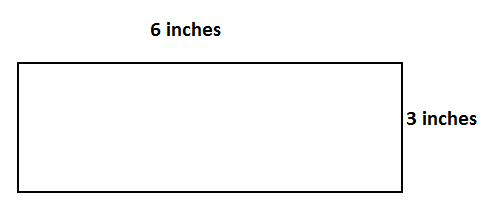Find the area.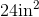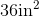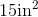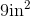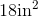Explanation:

To find the area of a rectangle, we will use the following formula: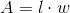where l is the length and w is the width of the rectangle.

Now, given the rectanglewe can see the length is 6in and the width is 3in. Knowing this, we can substitute into the formula.  So, we get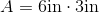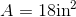### Example Question #52 : Rectangles

Find the area of a rectangle with a length of 16in and a width that is a quarter of the length.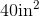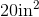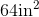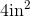Explanation:

To find the area of a rectangle, we will use the following formula:where l is the length and w is the width of the rectangle.

Now, we know the length of the rectangle is 16in.

We know the width is a quarter of the length.  So, we will divide the length by 4.  Therefore, the width is 4in.

So, we can substitute.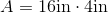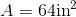### Example Question #53 : Rectangles

Find the area of a rectangle with a length of 16in and a width that is half the length.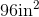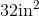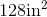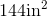Explanation:

To find the area of a rectangle, we will use the following formula:where l is the length and w is the width of the rectangle.

Now, we know the length of the rectangle is 16in.  We know the width is half the length.  Therefore, the width is 8in.  So, we can substitute.  We get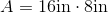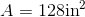### Example Question #111 : Quadrilaterals

Find the area of a rectangle with a length of 20in and a width that is a quarter of the length.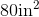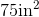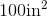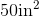Explanation:

To find the area of a rectangle, we will use the following formula:where l is the length and w is the width of the rectangle.

Now, we know the length of the rectangle is 20in.  We know the width is a quarter of the length.  So, we will divide by 4.  Therefore, the width is 5in.  So, we can substitute.  We get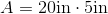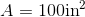### Example Question #211 : Geometry

Find the area of a rectangle with a length of 10in and a width that is half the length.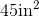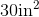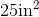Explanation:

To find the area of a rectangle, we will use the following formula:where l is the length and w is the width of the rectangle.

Now, we know the length of the rectangle is 10in.  We also know the width of the rectangle is half the length.  Therefore, the width is 5in.  So, we get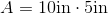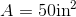### Example Question #56 : Rectangles

Find the area of a rectangle with a length of 16cm and a width that is a quarter of the length.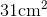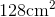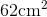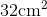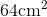Explanation:

To find the area of a rectangle, we will use the following formula:where l is the length and w is the width of the rectangle.

Now, we know the length of the rectangle is 16cm.  We also know the width of the rectangle is a quarter of the length.  To find the width, we will divide 16 by 4.  Therefore, the width the 4cm.

Knowing this, we will substitute into the formula.  We get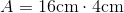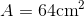### Example Question #57 : Rectangles

Find the area of a rectangle with the base of 12 and a height of 7.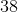Explanation:

The area of a rectangle is: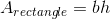Substitute the known lengths.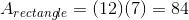The answer is:### Example Question #121 : Quadrilaterals

Find the area of the following figure: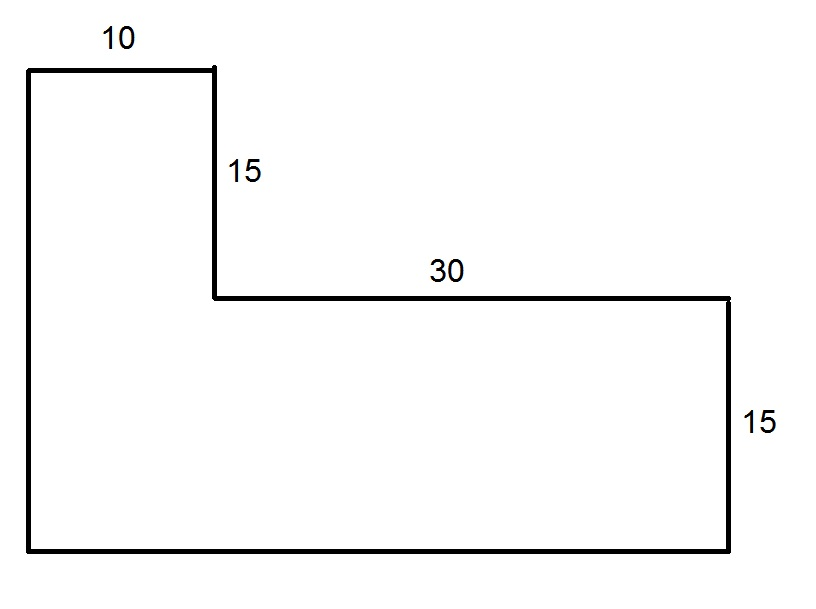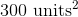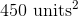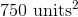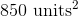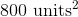Explanation:

Construct an additional segment as shown below.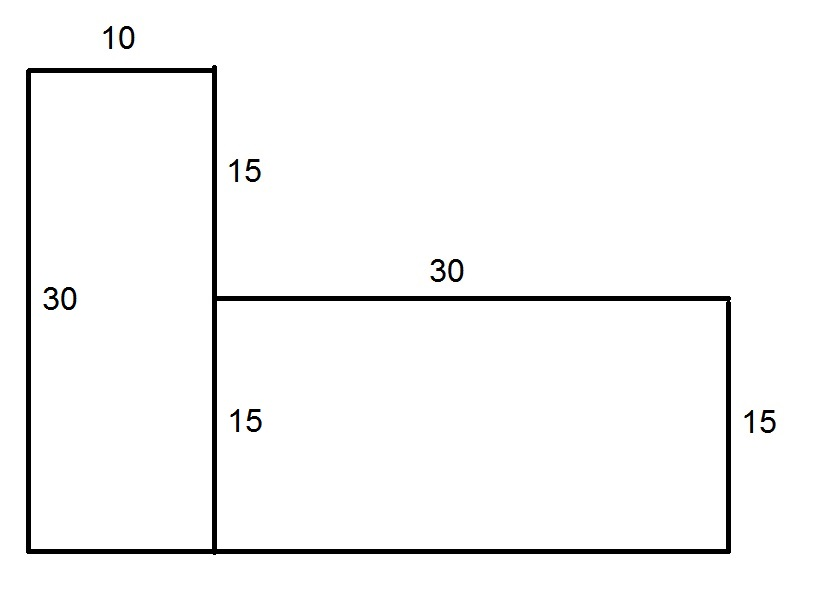Note that the figure can be divided into two rectangles. Also note that, since opposite sides of a rectangle are of the same length, we can fill in some sidelengths, as noted above.

The two rectangles each have areas that are the product of their lengths and widths: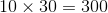and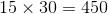Add these areas: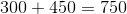, the area of the shape.

Area is written in square units; thus,1 2 3 4 6 Next →

### All ISEE Middle Level Math Resources